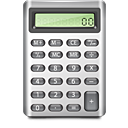## Math, numbers, calculations. The Math object in JavaScript.Well, other JavaScript objects we have discussed already, or we will discuss in next articles.

Today we will focus on numbers and the Number object in JavaScript, take a look closer at calculations and Math object in JavaScript.

#### JavaScript Number Object

It is a primary object to handle numbers, and has properties for numerical constants:

```var maxNumber = Number.MAX_VALUE;
var minNumber = Number.MIN_VALUE;
var infinity = Number.POSITIVE_INFINITY;
var minusInfinity = Number.NEGATIVE_INFINITY;
var isNotANmber = Number.NaN;
```

Methods of the Number object:

Scientific notation, digits parameter determines decimal places:

```number.toExponential([digits]);
```

Decimal notation:

```number.toFixed([digits]);
```

Determination of precision:

```number.toPrecision()
```

Example — precision after the decimal point:

```var number = new Number(51.967654321);
number.toPrecision(5); // result: 51.968
```

The next method returns a number as a string. The ‘base’ parameter specifies the number system (default, 2, 8, 16):

```number.toString([base])
```

The valueOf method returns a fundamental value represented by the Number object (stored value):

```number.valueOf()
```

Gets a float value from expression (string):

```number.parseFloat(string)
```

Extracts integer:

```number.parseInt(string[, radix])
```

where radix is a base (e.g. 2 — bin, 10 — dec).

If the base is not specified or is specified as 0, JavaScript assumes base 16, if the input string begins with “0x”.

Example — using parseInt method:

```parseInt("F", 16);
parseInt("17", 8);
parseInt("15", 10);
parseInt(15.99, 10);
parseInt("FXX123", 16);
parseInt(1111, 2);
parseInt("15*3", 10);
parseInt("12", 13);
```

All of the above examples return a result of 15.

The object has also properties: prototype and constructor, so can be used as instance constructor, for example:

```var num = new Number(123);
```

The situation is different in the case of the Math object.

#### Math object in JavaScript

All the methods and properties of the Math object are static. This means that they are available without an object instance, and for example such code:

var x = new Math();

is incorrect (causes TypeError).

Example — using Math object:

```alert(Math.abs(-123)); // 123

```

Properties of the Math object

– E – mathematical constant e (Euler’s constant, 2,71…)

– LN2 – the natural logarithm of 2 (0.693)

– LN10 – the natural logarithm of 10 (2.302)

– LOG2E – log base 2 of the E number (1.442)

– LOG10E – log base 10 of the E number (0.434)

– PI – mathematical constant PI (3.141593)

– SQRT1_2 – the square root of 1/2 (0.707)

– SQRT2 — the square root of 2 (1.414)

Methods of the Math object

– abs(number) – returns the absolute value of the number

– acos(number) – returns the arc cosine of a number (given in radians)

– asin(number) – returns the arcsine of a number (given in radians)

– atan(number) – returns the arctangent of a number (given in radians)

– atan2(y, x) – returns the arctangent of the quotient of the arguments

– ceil(number) – returns the smallest integer greater than or equal to (> =) given number

– cos(number) – returns the cosine of a number

– exp(x) – returns e (Math.E) raised to the power specified as an argument, equivalent of Math.pow (Math.E, x)

– floor(number) – returns the greatest integer less than or equal to (<=) given number – log(x) – returns the natural logarithm of x

– max([val1 [ , val2 [ , …]]]) – returns the largest number

– min([val1 [ , val2 [ , …]]]) – returns the smallest number

– pow(base, exponent) – returns the value of ‘base’ raised to the power of ‘exponent’

– random() – generates a pseudo-random value between 0 and 1

– round(number) – returns a number rounded to the nearest integer

– sin(number) – returns the sine of a number

– sqrt(number) – returns the square root of a number

– tan(number) – returns the tangent of a number

Some examples of the use below.

Example — rounding numbers in JavaScript:

```alert(Math.round(4.7)); // 5

// to two decimal places
alert(Math.round(27.451 * 100) / 100); // 27.45

// to one decimal
alert(Math.round(27.451 * 10) / 10); // 27.5

// to three decimal places
alert(Math.round(7.111111 * 1000) / 1000);  // 7.111

```

Note the need for multiplication of the number by 10, 100 or 1000, and then dividing the total by 10, 100 or 1000, as appropriate.

Example — random numbers in JavaScript:

```alert(Math.random());

```

Example — Min and Max:

```alert(Math.min(1,2,3));

```

Example — pow() and sqrt():

```var delta = Math.pow(b, 2) - (4 * a * c);

var sqrtDelta = Math.sqrt(delta);
```

#### Math in JavaScript — summary

Information from this article, and of course the knowledge of mathematics, are enough to efficiently perform calculations in JavaScript.

This is really useful, especially when writing games or programming more advanced operations on maps (e.g. Google Maps).